# 1 Introduction

任务驱动：解决对比学习效率低下的问题。【针对数据增强、判别器训练耗时】

本文工作：

• 如何构建锚点嵌入、正嵌入、负嵌入；
• 根据 Triplet loss 设计一种新损失函数；

本文对比学习思想：减小类内距离，增大类间距离。【类似聚类】

研究现状

• 无监督图表示学习（UGRL）中的对比学习：通常依赖于数据增强来生成输入内容（ input content）及其相关内容（related content），以实现 MI 最大化，从而导致训练过程的计算成本昂贵。
• 相关工作
• Deep Graph Infomax (DGI)最大化节点表示和图表示之间的互信息；
• Graphical Mutual Information (GMI)最大化输入图和输出图之间的互信息；
• GCA 通过各种数据增强，例如，属性掩蔽或边扰动，最大化两个视图每个节点之间的互信息；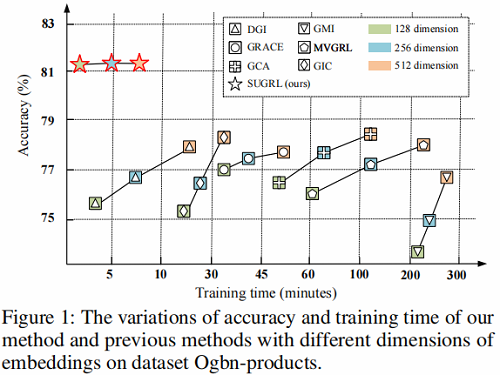对比学习工作存在的问题：数据增强、高维嵌入表示、对比损失函数设计带来的训练时间长问题，有关方法对比如 Figure 1 所示。

问题展开详述

• 数据增强：GRACEGCA 通过 remove edges 和 mask node 特性，以生成多个视图。因此，数据增强（包括视图生成和编码）的计算成本大约需要 20%-40% 的训练时间。
• 高维嵌入：通过提高嵌入的维数来提高表示质量，从而增加了训练时间。部分方法对维度敏感，如 DGIGMI 在 512 维空间上达到了它们最好的精度。
• 目标函数设计为判别器DGIMVGRL 使用一个判别器来测量节点嵌入和图嵌入的一致性（判别器为双线性判别器加 Sigmoid），花费约 10%-30% 的训练时间。【DGI、GMI、MVGRL、GIC 的判别器为双线性判别器】

# 2 Method

基于上述三个问题，本文提出 Simple Unsupervised Graph Representation Learning (SUGRL)，框架如 Figure 2 所示：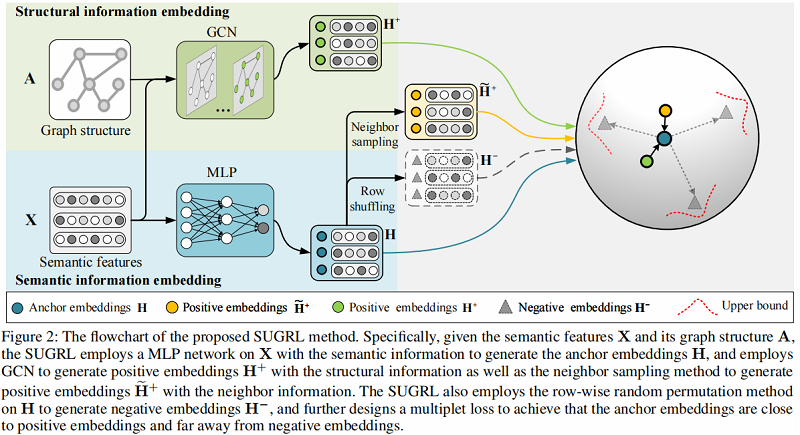方法步骤概述：

• 首先使用一个多层感知器(MLP)在带语义信息（semantic information）的输入 $X$ 上生成 Anchor embedding ；
• 接着基于 Graph structure $A$ 和 Semantic feature $X$ 使用 GCN 生成 Positive embedding。此外在 Anchor embedding 上通过 Neighbour sampling 生成另外一种 Positive embedding；
• 然后在 Anchor embedding 上通过 Row shuffling 生成一种 Negative embedding；
• 计算多重损失。

## 2.1 Anchor and negative embedding generation

Anchor 研究现状：大多将 node representation 和 graph summary 作为一个 Anchor 。

比如 ：

• DGI and MVGRL treat the graph summary as anchors, which is first convolved by GCN and then summarized by a readout function.
• GRACE and GCA regard the node embedding generated in one view as anchors.

上述产生的问题：大多需要经过 GCN 的传播，比较耗费时间。

Step1：本文生成锚嵌入（Amchor embedding）的方法是使用 MLP 作用在输入 $X$ 上，从而生成带语义信息的锚嵌入 。

$\begin{array}{l}\mathbf{X}^{(l+1)}=\text { Dropout }\left(\sigma\left(\mathbf{X}^{(l)} \mathbf{W}^{(l)}\right)\right) \quad\quad \quad\quad (1)\\ \mathbf{H}=\mathbf{X}^{(l+1)} \mathbf{W}^{(l+1)}\quad\quad\quad \quad\quad\quad\quad\quad\quad\quad\quad(2)\end{array}$

关于生成负嵌入，比较流行的方法如 DGI、GIC、MVGRL 采用的策略是：从原始图中得到一个被破坏的图，然后用 GCN 获得负嵌入。

Step2：本文生成负嵌入（Negative embedding）的方法是直接通过行打乱（row-shuffle） 去打乱锚嵌入。

$\mathbf{H}^{-}=\operatorname{Shuffle}\left(\left[\mathbf{h}_{1}, \mathbf{h}_{2}, \ldots, \mathbf{h}_{N}\right]\right)\quad\quad\quad(2)$

## 2.2 Positive embedding generation

现有的工作通常将结构信息 [ 数据增强后的视图为新的图结构 ] 视为正嵌入（Positive embedding），比如相关的工作有 DGI、MVGRL、GRACE、GCA、GIC 。

比如：

• 在 GCA 和 GRACE 中采用随机图数据增强；
• 在 MVGRL 中的图扩散；

本文生成了两种正嵌入（Positive embedding ）：

• structural embeddings
• neighbor embeddings

### 2.2.1 Structural information

Step3：为了获取带图的结构信息正嵌入，本文采用了广泛使用的 GCN 作为基编码器。

$\mathbf{H}^{+^{(l+1)}}=\sigma\left(\widehat{\mathbf{A}} \mathbf{H}^{+^{(l)}} \mathbf{W}^{(l)}\right)\quad\quad\quad\quad(4)$

注意，本文生成锚嵌入的 MLP 是和 这里的 GCN 共享权重矩阵 $W$ 的。

### 2.2.2 Neighbor information

Step4：为了得到具有邻居信息的 正嵌入，作者首先存储所有节点的邻居嵌入索引，然后对其进行抽样，然后计算样本的平均值。这样可以有效地获取节点的邻居信息：

$\widetilde{\mathbf{h}}_{i}^{+}=\frac{1}{m} \sum\limits _{j=1}^{m}\left\{\mathbf{h}_{j} \mid v_{j} \in \mathcal{N}_{i}\right\}\quad\quad\quad (5)$

其中

• $m$ 为采样邻居的个数；
• $\mathcal{N}_{i}$ 表示节点 $v_i$ 的一阶邻居（1-hop）集合；

总的来说，

• 结构嵌入（structural embedding）关注的是所有邻居信息，从 GCN 信息传递公式 $AXW$ 理解；
• 邻居嵌入（neighbor embedding）分别的邻居的某一部分，通过采样部分邻居信息；

因此，他们从不同的角度解释样本，从而将它们放在一起考虑，可能获得它们的互补信息。

## 2.3 Multiplet loss

DGI、GMI、MVGRL、GIC 使用双线性层作为判别器来区分正、负样本对，非常耗时间，具体对比可以参考 Figure 6

研究损失的目的：

• 拉近类内距离，加大类间距离；
• 减少泛化误差，减小泛化误差对 UGRL 来说也很重要，因为在训练过程中如果泛化误差小，可能会提高对比学习的泛化能力；

在 SUGRL中，本文以 Triplet loss 损失为基础，设计一个上限损失来避免使用除鉴别器。具体来说，每个样本的 Triplet loss 可以表示为：

$\alpha+d\left(\mathbf{h}, \mathbf{h}^{+}\right)<d\left(\mathbf{h}, \mathbf{h}^{-}\right)\quad\quad\quad(6)$

其中：

• $d(.)$ 是相似度测量方法，如 $\ell_{2}$ -norm distance；
• $\alpha$ 是非负值，代表着 positive 和 negative embeddings 的 "安全距离" ；

通过对所有负样本求和，$\text{Eq.6}$  扩展为：【类间分析】

$\mathcal{L}_{\text {triplet }}=\frac{1}{k} \sum_{i=1}^{k}\left\{d\left(\mathbf{h}, \mathbf{h}^{+}\right)^{2}-d\left(\mathbf{h}, \mathbf{h}_{i}^{-}\right)^{2}+\alpha\right\}_{+}\quad\quad\quad(7)$

其中

• $\{\cdot\}_{+}=\max \{\cdot, 0\}$
• $k$  代表负样本数

为了增加类间差异，作者拉大正负样本之间的距离，作者在两种 Positive embedding （考虑 Structural information 和 Neighbor information）上执行以下操作：

$\mathcal{L}_{S}=\frac{1}{k} \sum_{i=1}^{k}\left\{d\left(\mathbf{h}, \mathbf{h}^{+}\right)^{2}-d\left(\mathbf{h}, \mathbf{h}_{i}^{-}\right)^{2}+\alpha\right\}_{+}\quad\quad\quad(8)$

$\mathcal{L}_{N}=\frac{1}{k} \sum_{j=1}^{k}\left\{d\left(\mathbf{h}, \widetilde{\mathbf{h}}^{+}\right)^{2}-d\left(\mathbf{h}, \mathbf{h}_{j}^{-}\right)^{2}+\alpha\right\}_{+}\quad\quad\quad(9)$

分析上式的两种情况：

• Case 1：$d\left(\mathbf{h}, \mathbf{h}^{+}\right)^{2} \geq d\left(\mathbf{h}, \widetilde{\mathbf{h}}^{+}\right)^{2}$
• Case 2：$d\left(\mathbf{h}, \mathbf{h}^{+}\right)^{2}<d\left(\mathbf{h}, \widetilde{\mathbf{h}}^{+}\right)^{2}$

对于 Case 1：$d\left(\mathbf{h}, \mathbf{h}^{+}\right)^{2} \geq d\left(\mathbf{h}, \widetilde{\mathbf{h}}^{+}\right)^{2}$ ，那么即使 $\text{Eq.9}$ 为 $0$ ，$\text{Eq.8}$ 也不可能小于 $0$ （非负）。此时，我们可以认为 $\mathcal{L}_{S}$ 任然有效，$\mathcal{L}_{N}$  是无效的。因此，可以通过 $Eq.8$ 将锚点嵌入和负嵌入相推离，这样类间差异增大。与 Case 1 类似，Case 2 也可以扩大类间差异。

基于以上分析，Case 1 或 Case 2 都可以增大类间距离。特别是，如果其中一种无效，另一种仍将有效地进一步扩大类间距离。因此，$\text{Eq.8}$ 和 $\text{Eq.9}$ 可以从结构嵌入和邻域嵌入中获得互补的信息，从而能够扩大类间距离。

分析 $\text{Eq.7}$：【类内分析】

$\text{Eq.7}$ 保证的是 $d\left(\mathbf{h}, \mathbf{h}_{i}^{-}\right)^{2} - d\left(\mathbf{h}, \mathbf{h}^{+}\right)^{2}\ge \alpha$，这保证了类间距离，但忽略了类内距离（即 Anchor embedding 和 Positive embedding 的距离 ）。如果类内距离特别大，那么 $\text{Eq.7}$ 任然非负。在这种情况下，类内的变化可能会很大，但这并不有利于泛化误差的减少。

为了解决这个问题，作者通过以下目标函数研究了负对和正对的上界（即 $\alpha + \beta$）：

$\alpha+d\left(\mathbf{h}, \mathbf{h}^{+}\right)<d\left(\mathbf{h}, \mathbf{h}^{-}\right)<d\left(\mathbf{h}, \mathbf{h}^{+}\right)+\alpha+\beta\quad\quad\quad(10)$

其中：

• $\beta$  is a non-negative tuning parameter；

由式 $\alpha+d\left(\mathbf{h}, \mathbf{h}^{+}\right)<d\left(\mathbf{h}, \mathbf{h}^{-}\right)$ 可知类内距离有界；

由式 $d\left(\mathbf{h}, \mathbf{h}^{-}\right)<d\left(\mathbf{h}, \mathbf{h}^{+}\right)+\alpha+\beta$ 可知类间距离有界；

对所有负嵌入的损失加和后，提出的减少类内变化的上界损失定义如下：【理解这里的 $\text{min}$ 请结合 $\text{Eq.11}$ 和 $\text{Eq.10}$】

$\mathcal{L}_{U}=-\frac{1}{k} \sum\limits _{i=1}^{k}\left\{d\left(\mathbf{h}, \mathbf{h}^{+}\right)^{2}-d\left(\mathbf{h}, \mathbf{h}_{i}^{-}\right)^{2}+\alpha+\beta\right\}_{-}\quad\quad\quad(11)$

其中

• $\{\cdot\}_{-}=\min \{\cdot, 0\}$

注意到这里并没有考虑 neighbor information ，这是由于

• 每种信息都得到了相似的结果；
• 在实验中同时使用它们并不能显著提高模型的性能；

最后，将上述损失整合，可以表示为：

$\mathcal{L}=\omega_{1} \mathcal{L}_{S}+\omega_{2} \mathcal{L}_{N}+\mathcal{L}_{U}\quad\quad\quad(12)$

# 3 Experiments

8个基准数据集，包括3个引文网络数据集（即 Cora, Citeseer 和 Pubmed ）， 2个亚马逊销售数据集（即照片和计算机），3个大型数据集（即 Ogbn-arxiv、Ogbn-mag 和 Ogbn-products ）。

Baseline

1 种传统算法 DeepWalk， 2 种半监督学习算法（即 GCN 和 GAT），以及8种非监督学习算法( GAE，VGAE、DGI 、GRACE 、GMI 、MVGRL 和 GCA 、GIC 。

Table 1 和 Table 2 总结了所有方法在 8 个实际图结构数据集上的分类精度和执行时间。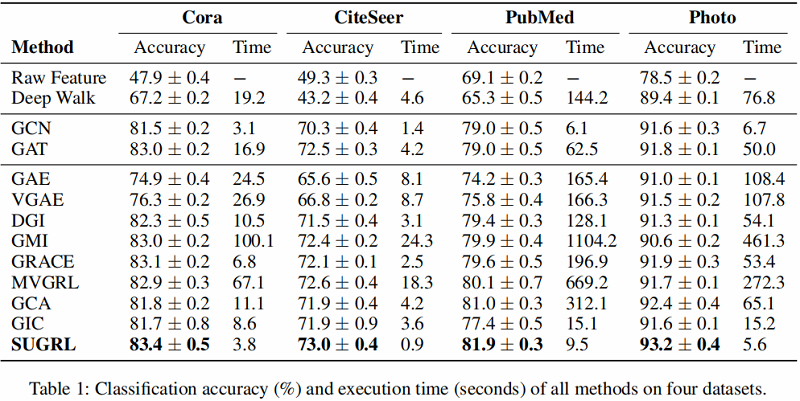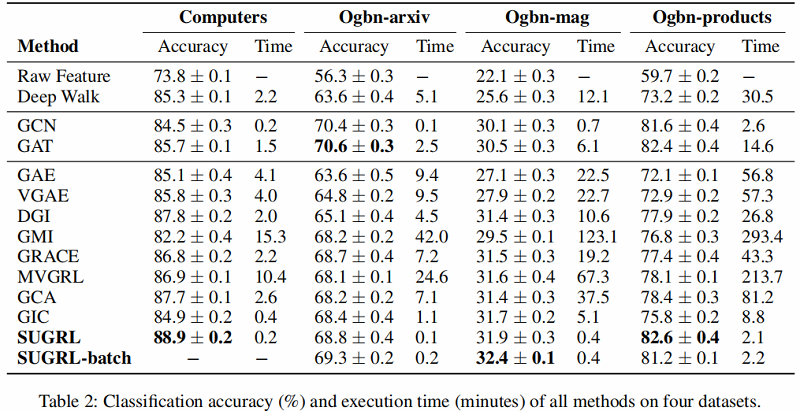结果分析：

• 首先，SUGRL在分类精度方面优于所有自监督方法（如DGI、GMI、GRACE、MVGRL、GIC和GCA)。例如，作者的方法与最差的方法DGI和最好的比较方法MVGRL相比，平均分别提高了4.0%和1.9%。与采用标签信息的半监督方法（GCN 和 GAT）相比，SUGRL也取得了更好的性能。
• 其次，作者的SUGRL的效率是最好的。在8个数据集上，与其他自监督方法相比，SUGRL 分别比最慢的比较方法 GMI 和最快的比较方法 GIC 平均快了 122.4 和 4.4 。

总之，作者的方法在几乎所有数据集上，在模型性能和执行时间方面，在节点分类方面都优于其他比较方法。原因可以总结如下。

• 首先，SUGRL综合考虑结构信息和邻域信息，生成两种正嵌入及其损失函数，这可以将负嵌入推离锚嵌入更远(即实现较大的类间变异)。
• 其次，SUGRL采用一个上界来保证正埋点和锚埋点之间的距离是有限的(即实现较小的类内变化)。
• 最后，SUGRL去掉了数据增大和鉴别器的步骤，大大减少了训练时间。最后，SUGRL可用于输出低维高质量的嵌入，在保持模型有效性的同时减少训练时间。

UGRL考虑三种信息，即语义信息、结构信息和邻居信息，生成两种具有对应对比损失的正对($\mathcal{L}_{S}$和$\mathcal{L}_{N}$)。为了验证框架中各成分的有效性，作者分别研究了结构信息、邻居信息和上界的有效性，以及对比损失中各成分的有效性。类内和类间距离之比的有效性。考虑到类内和类间距离的大小不同，将比率归一化为 $[0,1]$，在 Figure 3 的数据集Photo上报告类内与类间距离的比率。首先，与本文方法相比，没有结构或邻居信息的方法通常输出更大的比率(即更小的类间变异)。二是无上界的方法，与作者的方法相比，还会输出更大的比率(即更大的类内变量)。这样就可以验证结构信息、邻居信息或上界信息的有效性。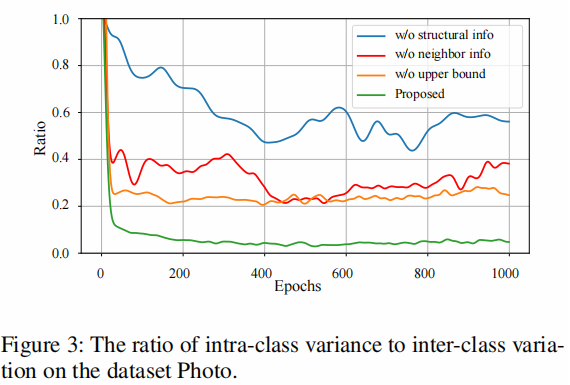Hyper-parameter analysis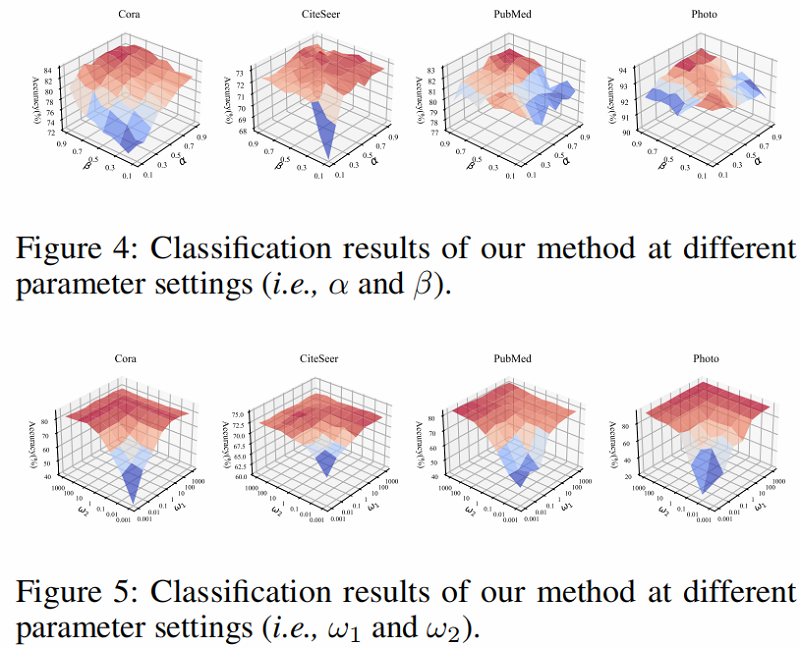Efficiency analysis# 4 Conclusion

在本文中，作者设计了一个简单的框架，即简单无监督图表示学习(SUGRL)，以实现有效和高效的对比学习。为了获得有效性，作者设计了两个三重组损失函数来探索结构信息和邻居信息之间的互补信息，以扩大类间的变化，以及一个上限损失来减少类内的变化。为了提高算法的效率，作者设计了一种去除GCN的生成锚点和负嵌入的方法，并从之前的图对比学习中去除数据增强和鉴别器。作者对各种真实世界的数据集进行了全面的实验，实验结果表明，作者的方法在准确性和可扩展性方面都优于目前最先进的方法。

补充：triplets loss 知识点

公式为：

$L=\max (d(a, p)-d(a, n)+\operatorname{margin}, 0)$

优化目标：拉近 $a$，$p$ 的距离，拉远 $a$，$n$ 的距离

• easy triplets : $L=0$  即 $d(a, p)+ margin <d(a, n)$，这种情况不需要优化，天然 $\mathrm{a}$，$\mathrm{p}$  的距离很近，$\mathrm{a}$，$\mathrm{n}$  的距离远；
• hard triplets: $d(a, n)<d(a, p)$，即 $a$，$p$ 的距离远；
• semi-hard triplets : $d(a, p)<d(a, n)<d(a, p)+ margin$，即 $\mathrm{a}$，$\mathrm{n}$  的距离靠的很近，但是有一个 $margin$ ；

# 修改历史

2021-03-25 创建文章

2022-06-10 修订文章

posted @ 2022-03-25 11:00  Learner-  阅读(381)  评论(0编辑  收藏  举报